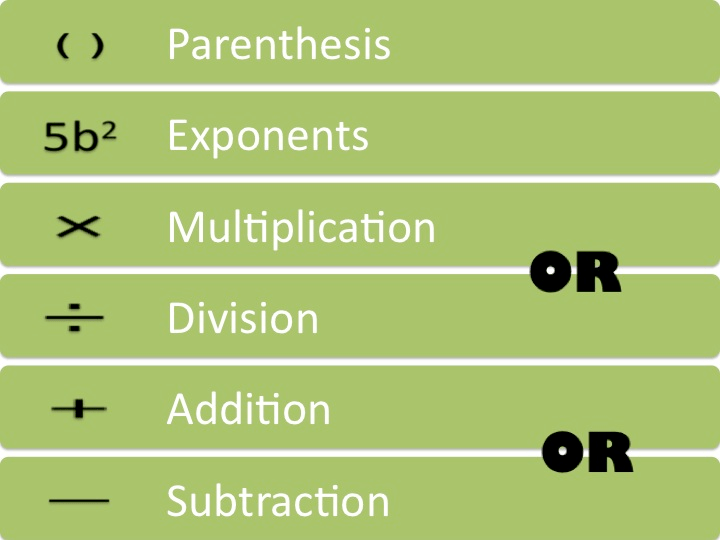# Order of Operations1. Perform operations in parentheses.
2. Find the values of numbers with exponents.
3. Multiply or divide from left to right in the order it appears in the problem.
4. Add or subtract from left to right in the order it appears in the problem.

A fun way to remember the order of operationsis the phrase ” Please Excuse MDear Aunt Sally.”   The first letter in each word can help you remember the order of operations.

## Why is this important?

2 x 32   = 2 x 9 = 18  without PEMDAS you could get 2 x 3 = 6 and square it to get 36!

2 + 3 x 15 = 47 without PEMDAS you could answer 5 x 15 = 75!

Following PEMDAS gets you the correct, expected answer.

Note: In Canada and the UK, they say brackets instead of parentheses. In the UK they say orders instead of exponents. “Brackets, Orders, Divide, Multiply, Add, Subtract”

(source – source links are NOT meant to be clicked/used.)